Courses

# Test: Diffusion Of Gases

## 22 Questions MCQ Test Chemistry for JEE | Test: Diffusion Of Gases

Description
This mock test of Test: Diffusion Of Gases for JEE helps you for every JEE entrance exam. This contains 22 Multiple Choice Questions for JEE Test: Diffusion Of Gases (mcq) to study with solutions a complete question bank. The solved questions answers in this Test: Diffusion Of Gases quiz give you a good mix of easy questions and tough questions. JEE students definitely take this Test: Diffusion Of Gases exercise for a better result in the exam. You can find other Test: Diffusion Of Gases extra questions, long questions & short questions for JEE on EduRev as well by searching above.
QUESTION: 1

### Direction (Q. Nos. 1-14) This section contains 14 multiple choice questions. Each question has four choices (a), (b), (c) and (d), out of which ONLY ONE option is correct. Q. 50 mL of H2 gas diffuse through a small hole from a vessel in 20 min. Time taken by 40 ml. of O2 gas to diffuse under similar conditions will be

Solution:

Volume of hydrogen = 50 mL; Time for diffusion (t) = 20 min and volume of oxygen = 40 mL. Rare of diffusion of hydrogen (r1) = 50/20 = 2.5 mL/min Rate of diffusion of oxygen (r2) = 40/t mL/min Since the molecular mass of hydrogen (M1) = 2 and that of oxygen (M2) = 32, therefore :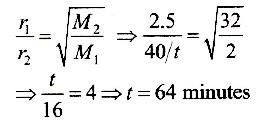QUESTION: 2

### It takes 26 s for 10 mL of H2 gas to effuse through a porous membrane, it takes 130 s for 10 mL of an unknown gas under identical conditions to effuse. Hence, molar mass of the unknown gas (in g mol-1) is

Solution:

The correct answer is Option B.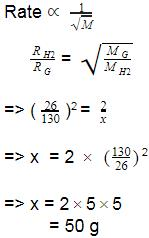QUESTION: 3

### 100 mL of O2 gas diffuse in 10 s. 100 mL of gas X diffuse in t s. Gas X and time t respectively can be

Solution:

t2/t1 = √M2/M1
t1 = 10 s, M1 = 32g, t2 = t and M2 = M
So we have t/10 = √M/32
Putting the values of options in the above relation, we gat the correct answer.
On putting values of option a.
2.5/10 = √(2/32)
¼ = √(1/16)
So,LHS = RHS

QUESTION: 4

Rate of diffusion of LPG (mixture of n-butane and propane) is 1.25 times faster than that of SO3. Hence, mole fraction of n-butane in LPG is

Solution:

rLPG / rSO3  =  √(80/ MLPG) = 1.25

Therefore, 80/M = (1.25)2

Therefore, MLPG  =  51.2

M =  (M1(butane ) X1 + M2(propane)X2)  / (X1 + X2)

51.2 = 58X1 + 44(1-X1) / 1

X1 ≈ 0.50

QUESTION: 5

Assume that the air in the space vehicle cabin consists of O2 with mole fraction, 0.20. if air contains both N2 and O2, then fraction of O2 that comes out the hole is

Solution:
QUESTION: 6

Consider a tube that has a frilled disk sealed in its centre so that gases can effuse through it in both directions. If the left hand side contains H2 at a pressure p and temperature T and the right hand side contains He at a pressure 2p and temperature T, then initial ratio of rate of effusion is

Solution:
QUESTION: 7

According to Graham’s law at a given temperature, the rates of diffusion rA / rB of gases A and B is given by (where, p and M are partial pressure and molar masses)

[IIT JEE 1998, 2011]

Solution:

According to Graham's law,

We have: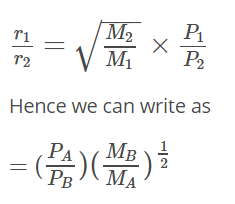QUESTION: 8

If helium and methane are allowed to diffuse out of the container under the similar conditions of temperature and pressure, then the ratio of rate of diffusion of helium to methane is

[IIT JEE 2005]

Solution: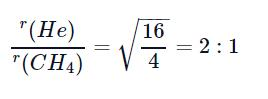QUESTION: 9

Sulphur dioxide and oxygen were allowed to diffuse through a porous partition. 20 dm3 of SO2 diffuses through the porous partition in 60 s. The volume of O2 (in dm3) which diffuses in 30 s will be (atomic mass of sulphur = 32 u)

[JEE Main 2014]

Solution:
QUESTION: 10

A vessel contains 0.5 mole each of SO2, H2 and CH4.  Its outlet was made open and closed after some time. Thus, order of partial pressure inside the vessel will be

Solution:

From Graham's law of diffusion, the rate of diffusion of a gas through a slit or any outlet or inlet in a container, is inversely proportional to the molecular weight of the gas.

So , for a definite time (say t), SO2 will diffuse in the least amount through the outlet as it has highest mass among the three. Similarly the next in the increasing order will be CH4 and finally the last one is H2 will diffuse in the most amount.

Thus, the final amount of gases in the container are in the order SO2 > CH4 > H2.

It's clear that more the amount of the gas, more is its partial pressure.

So, the correct order is P(SO2) > P(CH4) > P(H2)

QUESTION: 11

Consider the following pairs of gases A and B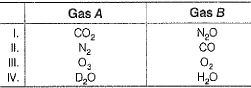Q. Thus, relative rates of diffusion of gases A and B is in the order

Solution:

r1 = √(44/44 = 1
r2 = √(44/44) = 1
r3 = √(32/48) = 0.81
r4 = √18/20 = 0.94
So the order is
III < IV < I = II

QUESTION: 12

1 mole of helium and 3 moles of N2 exert a pressure of 16 atm. Due to a hole in the vessel in which mixture is placed, mixture leaks out. Thus, composition of the mixture effusing out initially is

Solution:

Here, total moles = 4+1 = 5, total pressure = 20 bar
Partial pressure of He = 4/5×20 = 16 bar
Partial pressure of CH4 = 1/5×20 = 4 bar
Ratio of rate of diffusion of He and CH4 will be
rHe/rCH4 = (pHe / pCH4)(MCH4 / MHe
= (16/4)×(16/4)½
= 8
So, the rate of effusion of He is 8 times higher than CH4. Therefore Initial mixture must contain the molar ratio of He:CH4 = 8:1

QUESTION: 13

For 10 min each, at 27°C from two identical holes, nitrogen and an unknown gas are leaked into a common vessel of 3 L capacity. The resulting pressure is 4.18 bar and the mixture contains 0.4 mole of nitrogen gas. Thus, molar mass of the unknown gas is (g mol-1)

Solution:

V = 3L , T = 300 K , R = 0.0821 Lit atmJ/K mol, P = 4.18 Bar
So, total moles of solution n =PV/RT  = 4.18 x 3 /(0.0821 x 300)  = 0.5 moles
As moles of N2 = 0.4
Hence, no. of moles of unknown gas = 0.5 -0.4 = 0.1 moles
Now, from Graham's law of diffusion,
Rate of effusion of nitrogen (rN2) = no. of moles/ time taken = 0.4/ 10 = 0.04 mol/min
Rate of effusion of unknown gas (runknown) =0.1/10 = 0.01 mol/min
Since, rate of diffusion ∝ 1/√Molar mass
rN2/runknown = √Munknown/MN2
√(Munknown/MN2) = 4
Munknown = 16×28 = 448 gm/mol

QUESTION: 14

At the start of an experiment, one end of a U-tube of 6 mm glass tubing is immersed in concentrated ammonia solution and the other end in concentrated hydrochloric acid. At the point in the tube where vapours of ammonia and hydrochloric acid meet, white cloud is formed. At what fraction of the distance along the tube from the ammonia solution, the white cloud first form ?

Solution:

d/6-d = √(36.5/17)  (d is distance from ammonia end, rate = distance/time both have same time)
d/6-d = 1.46
d = 3.56
Fraction = 3.56/6  = 0.593

*Multiple options can be correct
QUESTION: 15

Direction (Q. Nos. 15-17) This section contains 3 multiple choice questions. Each question has four choices (a), (b), (c) and (d), out of which ONE or  MORE THANT ONE  is correct.

Q. Rates of diffusion of two gases A and B are rA and rB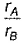is equal to

Solution:

Rate of diffusion ∝ 1/√M
As mass and density are proportional. So, rate of diffusion ∝ 1/√D
Rate ∝ pressure
And rate = volume /Time.
Considering these, all options are correct.

*Multiple options can be correct
QUESTION: 16

Ratio of the rate of diffusion of He to H2 at 0°C is same

Solution:
*Multiple options can be correct
QUESTION: 17

100 mL of H2 gas diffuse in 10 s. X mL of O2 diffuses in t s. Thus, X and t respectively are

Solution:

rH2 = 100/10 = 10
rO2 = x/t
rH2/rO2 = √MO2/MH2
10/rO2 = 4
rO2 = 5/2
So, all options except c give us 5/2. So, option a, b and d are correct.

QUESTION: 18

Direction (Q. Nos. 18-21) This section contains 2 paragraph, wach describing theory, experiments, data etc. three Questions related to paragraph have been given.Each question have only one correct answer among the four given options (a),(b),(c),(d).

Passage I

Uranium content of 235U in uranium ore is enriched from a mixture of 235UF6 and 238UF6 by effusion method (F - 19.0 g mol-1).

Q. Enrichment factor is

Solution:

The correct answer is option A.
The first step is to calculate the molar mass of UF6 containing 235U and 238U. For the success of the separation method, fluorine consists of a single isotope of atomic mass 18.998. The molar mass of 235UF6 is
234.04 + (6)(18.998) = 349.03 g/mol
The molar mass of 238UF6 is
238.05 + (6)(18.998) = 352.04 g/mol
The difference is only 3.01 g/mol (less than 1%). The ratio of effusion rates can be calculated from Graham’s Law using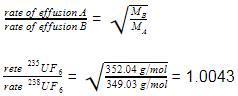QUESTION: 19

For permanent gases diffusivity the order is _________ sq.m/s

Solution:

The gas can become permeable to certain substance if it is in an order of 10-10.

QUESTION: 20

Passage II

X and Y are two volatile liquids with molar weights of 10 g mol-1 and 40 g mol-1 respectively. Two cotton plugs, one soaked in X and the other soaked in Y, are simultaneously placed at the ends of the tube of length l = 24 cm , as shown in the figure. The tube is filled with an inert gas at 1 atm pressure and temperature of 300 K. Vapours of X and Y react to form a product which is first formed at a distance, d cm from the plug soaked in X. Take X and Y to have equal molecular diameters and assume ideal behaviour for the inert gas and two vapours.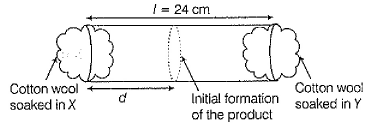Q. The value of d in cm (shown in the figure), as estimated from Graham’s law is

Solution:

From Graham's law of diffusion, r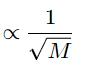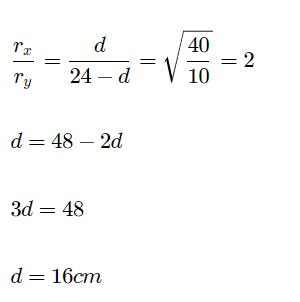QUESTION: 21

Passage II

X and Y are two volatile liquids with molar weights of 10 g mol-1 and 40 g mol-1 respectively. Two cotton plugs, one soaked in X and the other soaked in Y, are simultaneously placed at the ends of the tube of length l = 24 cm , as shown in the figure. The tube is filled with an inert gas at 1 atm pressure and temperature of 300 K. Vapours of X and Y react to form a product which is first formed at a distance, d cm from the plug soaked in X. Take X and Y to have equal molecular diameters and assume ideal behaviour for the inert gas and two vapours.Q. The experimental value of d is found to be smaller than the estimated value obtained by using Graham's law. This is due to

Solution:

The general formula for mean free path(λ) is
λ = RT/√2 π d2NAp
(d = diameter of molecule, p = pressure inside vessel)
Since d and p are the same for both gases, ideally their λ are the same. Hence it must be the higher drift speed of X due to which it is facing more collisions per second with the inert gas in comparison to gas Y. Hence X faces more resistance with the inert gas than Y and hence covers lesser distance than that predicted by Graham's law.

*Answer can only contain numeric values
QUESTION: 22

Direction (Q. No. 22) This section contains 1 questions. when worked out will result in an integer from 0 to 9 (both inclusive).

Q. At 1200°C, mixture of Cl2 and Cl-atoms (both in gaseous state) effuses 1.16 times as fast as a gas of molar mass 87.6 g mol-1, under identical conditions. What is the percentage dissociation of Cl2 into Cl-atoms ?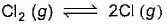Solution:

The rate of effusion of a gas is inversely proportional to its molecular weight.
r∝1/√M
Let x be the average molecular weight of a mixture of Cl2 and Cl atoms.The molecular weight of krypton is 87.6.
r(mixture ofCl2 and Cl atoms)/rX =√(MX/M(mixture of Cl2 and Cl atoms))
Substitute values in the above expression, we get:
1.16=√(87.6/x)
or x=65.37
Also, 65.37 = [(100−y)×71+y×35.5]/100
or y = 15.8
So, in 1000 moles species 842 moles is Cl2 molecules and 158 moles is  Cl atom.
So, initial moles of Cl2
=842+185/2
So, % fraction dissociated
185/2/(842+185/2)×100=9.89 % = 9%